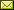## Automatic Repair of Overflowing Expressions with Abstract Interpretation

 Francesco Logozzo (Microsoft Research, Redmond, USA) Matthieu Martel (Université de Perpignan Via Domitia & LIRMM, UMR 5506, Montpellier, France)

 We consider the problem of synthesizing provably non-overflowing integer arithmetic expressions or Boolean relations among integer arithmetic expressions. First we use a numerical abstract domain to infer numerical properties among program variables. Then we check if those properties guarantee that a given expression does not overflow. If this is not the case, we synthesize an equivalent, yet not-overflowing expression, or we report that such an expression does not exists. The synthesis of a non-overflowing expression depends on three, orthogonal factors: the input expression (e.g., is it linear, polynomial,... ?), the output expression (e.g., are case splits allowed?), and the underlying numerical abstract domain - the more precise the abstract domain is, the more correct expressions can be synthesized. We consider three common cases: (i) linear expressions with integer coefficients and intervals; (ii) Boolean expressions of linear expressions; and (iii) linear expressions with templates. In the first case we prove there exists a complete and polynomial algorithm to solve the problem. In the second case, we have an incomplete yet polynomial algorithm, whereas in the third we have a complete yet worst-case exponential algorithm.

In Anindya Banerjee, Olivier Danvy, Kyung-Goo Doh and John Hatcliff: Semantics, Abstract Interpretation, and Reasoning about Programs: Essays Dedicated to David A. Schmidt on the Occasion of his Sixtieth Birthday (Festschrift for Dave Schmidt), Manhattan, Kansas, USA, 19-20th September 2013, Electronic Proceedings in Theoretical Computer Science 129, pp. 341–357.
Published: 19th September 2013.

 ArXived at: http://dx.doi.org/10.4204/EPTCS.129.21 bibtex PDF
References in reconstructed bibtex, XML and HTML format (approximated).
 Comments and questions to:eptcs@eptcs.org For website issues:webmaster@eptcs.org